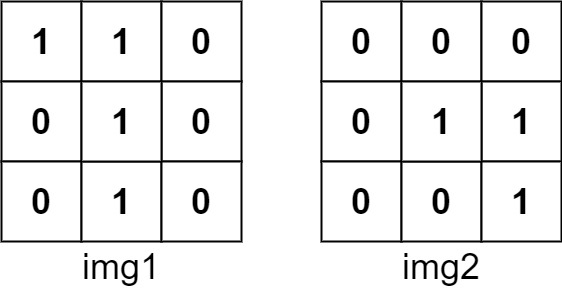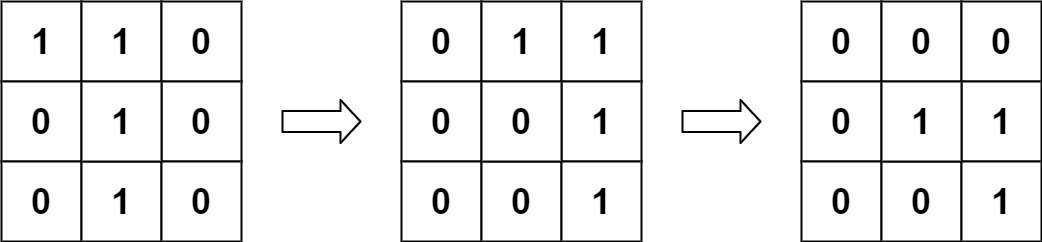## Algorithm

Problem Name: 835. Image Overlap

You are given two images, `img1` and `img2`, represented as binary, square matrices of size `n x n`. A binary matrix has only `0`s and `1`s as values.

We translate one image however we choose by sliding all the `1` bits left, right, up, and/or down any number of units. We then place it on top of the other image. We can then calculate the overlap by counting the number of positions that have a `1` in both images.

Note also that a translation does not include any kind of rotation. Any `1` bits that are translated outside of the matrix borders are erased.

Return the largest possible overlap.

Example 1:```Input: img1 = [[1,1,0],[0,1,0],[0,1,0]], img2 = [[0,0,0],[0,1,1],[0,0,1]]
Output: 3
Explanation: We translate img1 to right by 1 unit and down by 1 unit.The number of positions that have a 1 in both images is 3 (shown in red).```

Example 2:

```Input: img1 = [], img2 = []
Output: 1
```

Example 3:

```Input: img1 = [], img2 = []
Output: 0
```

Constraints:

• `n == img1.length == img1[i].length`
• `n == img2.length == img2[i].length`
• `1 <= n <= 30`
• `img1[i][j]` is either `0` or `1`.
• `img2[i][j]` is either `0` or `1`.

## Code Examples

### #1 Code Example with Java Programming

```Code - Java Programming```

``````
class Solution {
public int largestOverlap(int[][] img1, int[][] img2) {
List> nonZeroCoordinates1 = getNonZeroCoordinates(img1);
List> nonZeroCoordinates2 = getNonZeroCoordinates(img2);
int maxOverlap = 0;
Map, Integer> groupCount = new HashMap<>();
for (Pair c1 : nonZeroCoordinates1) {
for (Pair c2 : nonZeroCoordinates2) {
Pair vector = new Pair(
c2.getKey() - c1.getKey(), c2.getValue() - c1.getValue());
groupCount.put(vector, groupCount.getOrDefault(vector, 0) + 1);
maxOverlap = Math.max(maxOverlap, groupCount.get(vector));
}
}
return maxOverlap;
}

private List> getNonZeroCoordinates(int[][] arr) {
List> coordinates = new ArrayList<>();
for (int i = 0; i < arr.length; i++) {
for (int j = 0; j < arr.length; j++) {
if (arr[i][j] == 1) {
}
}
}
return coordinates;
}
}
``````
Copy The Code &

Input

cmd
img1 = [[1,1,0],[0,1,0],[0,1,0]], img2 = [[0,0,0],[0,1,1],[0,0,1]]

Output

cmd
3

### #2 Code Example with Javascript Programming

```Code - Javascript Programming```

``````
const largestOverlap = function(A, B) {
const N = A.length
const count = constructMatrix(2*N, 2*N, 0)
for (let i = 0; i < N; i++) {
for (let j = 0; j < N; j++) {
if (A[i][j] === 1) {
for(let ii = 0; ii < N; ii++) {
for(let jj = 0; jj < N; jj++) {
if(B[ii][jj] === 1) {
count[i-ii+N][j-jj+N] += 1
}
}
}
}
}
}
let ans = 0

for(let row of count) {
for(let v of row) {
ans = Math.max(ans, v)
}
}
return ans
};

function constructMatrix(row, col, init = 0) {
const matrix = []
for(let i = 0; i < row; i++) {
matrix[i] = []
for(let j = 0; j < col; j++) {
matrix[i][j] = init
}
}
return matrix
}

console.log(largestOverlap([[1,1,0],
[0,1,0],
[0,1,0]],[[0,0,0],
[0,1,1],
[0,0,1]]))
``````
Copy The Code &

Input

cmd
img1 = [[1,1,0],[0,1,0],[0,1,0]], img2 = [[0,0,0],[0,1,1],[0,0,1]]

Output

cmd
3

### #3 Code Example with Python Programming

```Code - Python Programming```

``````
class Solution:
def largestOverlap(self, A, B):
n, shift, rn = len(A), range(-1 * len(A) + 1, len(A)), range(len(A))
return max(sum(A[i][j] and B[i + v][j + h] for i in rn for j in rn if 0 <= i + v < n > j + h >= 0) for h in shift for v in shift)
``````
Copy The Code &

Input

cmd
img1 = [], img2 = []

Output

cmd
1

### #4 Code Example with C# Programming

```Code - C# Programming```

``````
using System;

namespace LeetCode
{
public class _0835_ImageOverlap
{
public int LargestOverlap(int[][] img1, int[][] img2)
{
int count = 0, N = img1.Length;
var counts = new int[N * 2, N * 2];
for (int i = 0; i < N; i++)
for (int j = 0; j < N; j++)
{
if (img1[i][j] == 0)
continue;

for (int m = 0; m < N; m++)
for (int n = 0; n < N; n++)
{
if (img2[m][n] == 0)
continue;

count = Math.Max(count, ++counts[N + i - m, N + j - n]);
}
}

return count;
}
}
}
``````
Copy The Code &

Input

cmd
img1 = [], img2 = []

Output

cmd
1